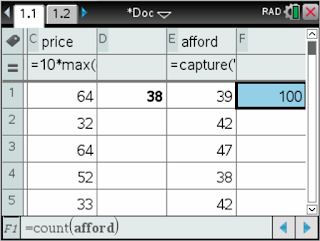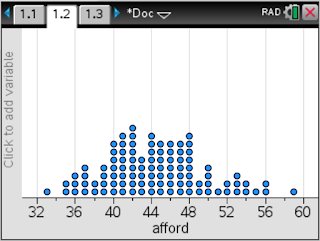## The problem.

This problem was introduced to me by Allan Rossman at the Simulating at Serenbe workshop last summer. The problem goes like this...

Suppose that you have only 50 cents in your pocket and you want to buy an ice cream cone.  The owner of the ice cream shop offers a random price determined as follows: You roll a pair of fair, six-sided dice, and the price is the larger number followed by the smaller number (in cents).  We will approximate, and then determine, the probability that you’ll be able to afford the ice cream cone.

Allan used a three-step approach to these problems that I would like to incorporate into as much as I can in stats. His approach to these problems involved intuition, simulation, and mathematics. Begin by getting a feel for the problem and the probability via your intuition (which is often wrong), then set out to conduct some sort of a simulation to get a better feel for the probability (Allan's choice was R, but I will share a GeoGebra simulation and an Nspire simulation and program), then tackle the mathematics of computing the probability (which in this problem, is more of listing all the outcomes in the sample space).

### Intuition

Before you jump into the problem, think about some specific prices that you could possibly pay for a cone. You could pay 43 cents when the owner rolls a 4 and a 3. How likely is this? What about paying 21 cents? How likely would it be to pay 23 cents (trick question)? What's the largest amount you would be asked to pay (regardless if you can afford it)? What's the smallest amount?

### Simulation

#### TI NspireCAS Simulation

I would want my students to actually roll some dice to begin with as a transition from intuition to simulation. Roll some dice, create some dot plots by hand as a class. I would then help them set up a spreadsheet on their Nspires. Since every AP Stats student will be loaned a TI NspireCAS this year (quite excited), most of the stat stuff you will see will involve the Nspire. If my school buys it, I am going to use it.

Here is a spreadsheet simulating 100 rolls of the dice, computing the price, and counting the number of prices less than 50 cents.Randint(1,6,100) to generate 100 random rolls of an imaginary die.10*max(die1,die2) + min(die1,die2) computes the price for the two rolls

Here is a distribution of 100 simulated prices.100 prices by 100 simulated rolls of the dice.

I am interested in how many prices are less than 50 cents, so I will keep track of this in another cell in the spreadsheet.countif(price,?<50) counts the number of values in the price column that are less than 50.

By storing this number, I can use automatic data capture to insert these values into another list.capture(aff,1) allows me to automatically capture the number of prices less than 50 in a list when I press ctrl+r on the handheld

I can then generate a list of the number of prices out of each 100 simulations that are less than 50 cents.count(afford) allows me to keep track of how many trials I have run. There are 100 data values in the afford list.

A dot plot of these numbers somewhere between 40 and 48 times out of 100 I will be able to afford an ice cream cone.Here are the number of prices that I can afford out of 100 simulations of 100 simulated rolls of the dice.

As soon as the students ask, "Is there a way to just press ctrl+r and have it do all this at one time?" they are ready to be introduced to writing a simple TI-Basic program that runs this simulation.

#### GeoGebra Simulation

Here is a GeoGebra simulation of rolls of the dice. This is pretty straight forward.

#### Desmos Activity Builder

Last week I was invited to attend the Desmos Fellows Weekend. Spending the weekend out there really got me going with the Desmos Activity Builder and the Computation Layer. Here is a Desmos Activity based upon this problem:  https://student.desmos.com?prepopulateCode=tjbeah

One of my goals for this year (besides trying to blog again) is to create Desmos Activities that accompany the Advanced Quantitative Reasoning text I use with some of my Seniors who are not in AP Stats.

### Teaching factoring without teaching factoring

I haven't taught Algebra 1 in a few years. But I was motivated to write something down in response to this thread, and in particular, Be...